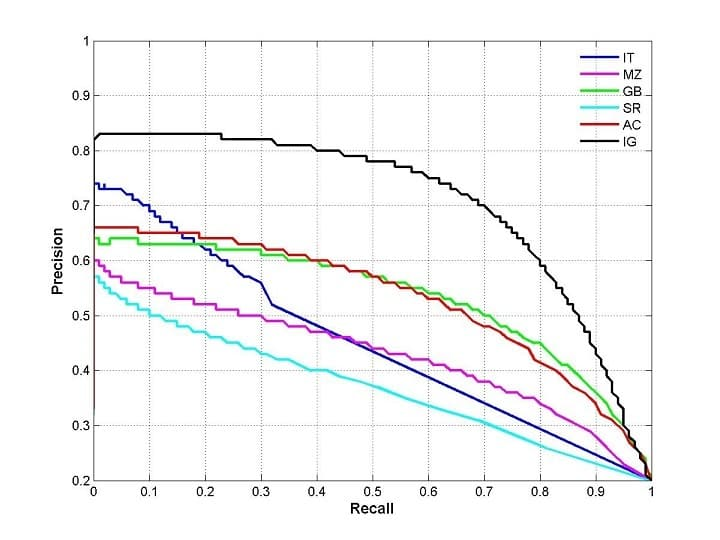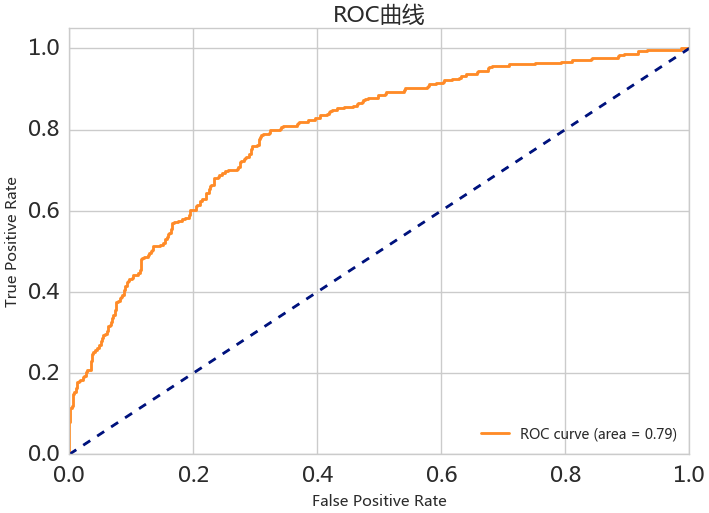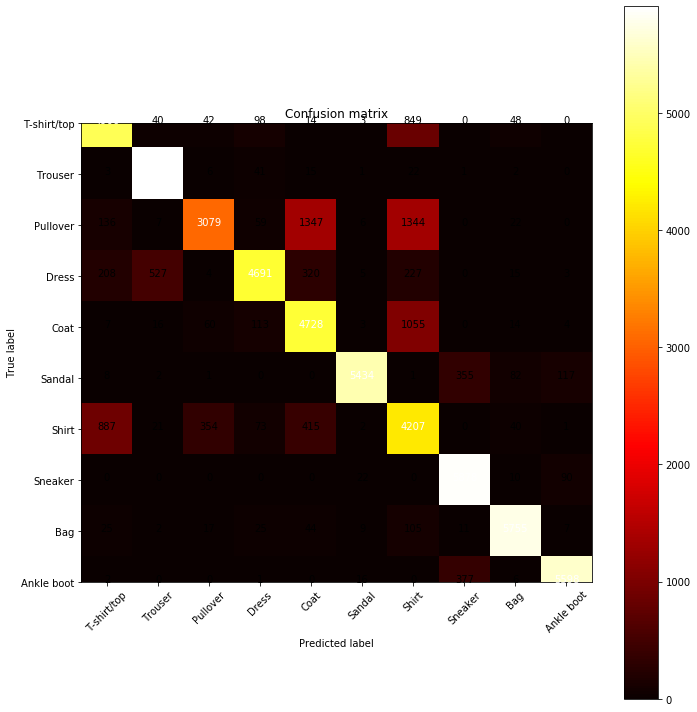# 数据集及其拆分

Iris（鸢尾花）数据集 为例，这个数据集包括三种花：山鸢尾、变色鸢尾、维吉尼亚鸢尾，我们根据 花萼（sepal）和 花瓣（petal）的长宽 对鸢尾花进行分类。• Features
• Sepal Length
• Sepal Width
• Petal Length
• Petal Width
• Class Label

1. 留出法（Hold-Out Method），将数据集随机地分为两组

• 优点是处理简单；缺点是性能评估结果依赖数据地拆分情况，不够稳定。
2. K折交叉验证，将数据集分为 K 份（K 一般取 5/10），每次用其中一个做测试集，其他做训练集，得到 K 个评价，取均值后得到最终评价结果。（PS. 每次训练都是从 0 开始，而不是从上一次开始）

• 优点是提高了评估结果地稳定性
3. 分层抽样策略（Stratified k-fold） 在 K折交叉验证的基础上，使得每一份内各个类型数据的比例和原始数据集中各个类型的比例相同。

# 分类问题

TP FN P
FP TN N

$\text{accuracy}=\frac{TP+TN}{P+N}$

$\text{precision}=\frac{TP}{TP+FP}$

$\text{recall}=\frac{TP}{P}$P-R 曲线的绘制方法如下：模型输出的是每个样本属于正类的概率，我们将所有概率排序，然后依次取每个概率作为阈值，规定大于阈值的为正类，这样就能计算出 P-R 曲线上的一点。取完所有的阈值后，就能得到 P-R 曲线。

$F_{\beta-\text{score}} = \frac{1}{\frac{\beta^2}{(1+\beta^2)R}+\frac{1}{(1+\beta^2)P}}\\=\frac{(1+\beta^2)\cdot P \cdot R}{\beta^2 \cdot P + R}$

$\beta$ 为重要程度，大于 0。当上式中的 $\beta$ 值取 $1$ 时，称为 $F_1$，表示两个同等重要。ROC 与 PRC 一样，也可根据所围成的面积 ROC-AUC 来评估模型的效果，面积越大，效果越好。同时 ROC 的画法和 PRC 一样。